Courses

# NCERT Solutions - Motion, Class 9, Science | EduRev Notes

## Class 9: NCERT Solutions - Motion, Class 9, Science | EduRev Notes

The document NCERT Solutions - Motion, Class 9, Science | EduRev Notes is a part of the Class 9 Course NCERT Textbooks (Class 6 to Class 12).
All you need of Class 9 at this link: Class 9

Page No. 100

Q1. An object has moved through a distance. Can it have zero displacements? If yes, support your answer with an example.
Ans: Yes, an object can have zero displacements even if it has moved through a distance.
Example: If an athlete runs around a circular path of radius 'r' and comes back to the initial point, then distance covered = 2πr, displacement = zero.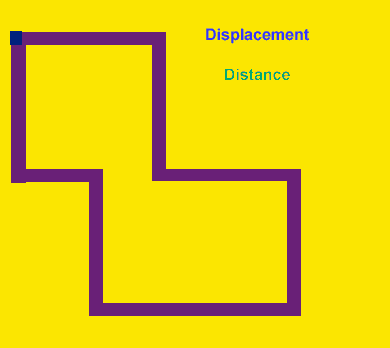Q2. A farmer moves along the boundary of a square field of side 10 m in 40s. What will be the magnitude of displacement of the farmer at the end of 2 minutes 20 seconds from his initial position?
Ans: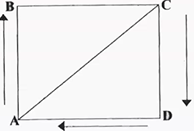Distance covered by farmer in 40 s = 40 m, i.e. perimeter of the square field.
If he completes one round in 40 s, then in 2 min 20s, i.e. 140s, he completes 140/40 = 3.5 rounds.
Thus, if he starts from point B (see figure), and reaches point D.
Displacement, BD = length of diagonal
Diagonal of square = side √2 = 10√2 = 10 x 1.414 = 14.14 m

Q3. Which of the following is true for displacement?
(a) It cannot be zero.
(b) Its magnitude is greater than the distance travelled by the object.
Ans:

(a) False; because the displacement is zero if an object returns to its initial position after completing the trip.
(b) False; the displacement always has equal or less magnitude than the distance travelled.

Page No. 102

Q1. Distinguish between speed and velocity.
Ans: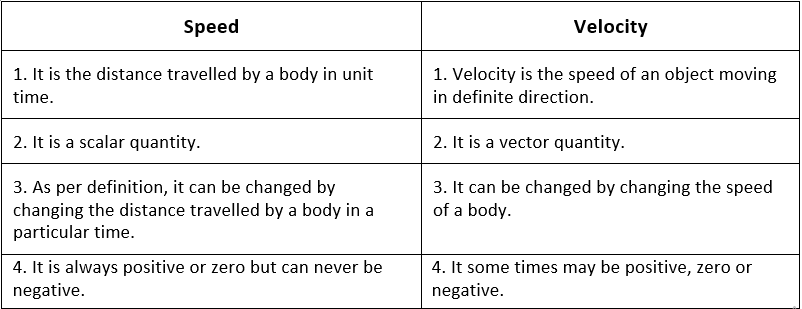Q2. Under what condition(s) is the magnitude of the average velocity of an object equal to its average speed?
Ans: When the object travels along a straight-line path,
i.e. Distance travelled = Displacement
⇒ Average speed = Average velocity.

Q3. What does the odometer of an automobile measure?
Ans: The odometer of an automobile measures the distance covered by it.

Q4. What does the path of an object look like when it is in uniform motion?
Ans: The path of the object will be a straight line at the instant of measurement when it is in uniform motion.

Q5. During an experiment, a signal from a spaceship reached the ground station in five minutes. What was the distance of the spaceship from the ground station? The signal travels at the speed of light, which is 3 × 108 ms-1.
Ans: Speed of signal = 3 × 108 ms-1
Time in which signal reaches ground = 5 min = 5 × 60 = 300 s
Distance of spaceship from the ground level = speed × time = 3 × 108 × 300 = 9 × 1010 m

Page No. 103

Q1. When will you say a  body is in
(i) Uniform acceleration (ii) Non-uniform acceleration?
Ans:

(i) If an object travels in a straight line and its velocity increases or decreases by equal amounts in equal intervals of time, then the body is said to be in uniform acceleration.
Example: The motion of a freely falling body.

(ii) If an object travels in a straight line and its velocity changes by unequal amounts in equal intervals of time, then the body is said to be in non-uniform acceleration.
Example: If a car is travelling along a straight road and passes through a crowd, suffers an unequal change in velocity, in equal intervals of time.

Q2. A bus decreases its speed from 80 km h−1 to 60 km h−1 in 5 s. Find the acceleration of the bus.

Ans: We know, 1km = 1000m ; 1hr = 3600s

Given, Initial speed of the bus,u  = 80 kmh-1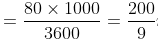ms-1

The final speed of the bus,v = 60 kmh-1=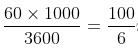ms-1

Time is taken, t= 5s

We know, v = u+at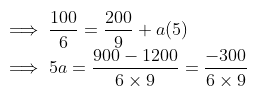= -1.11 ms-2

The negative sign implies retardation.

Therefore, the acceleration of the bus is -1.11 ms-2

Or, the retardation(de-acceleration) of the bus is 1.11 ms-2

Q3. A train starting from a railway station and moving with uniform acceleration attains a speed of 40 km/h in 10 minutes. Find its acceleration.
Ans:  Initial velocity, u = 0 km h-1; Final velocity, v = 40 kmh-1
Time, t = 10 min = 1/6 h
Acceleration,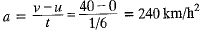Page No. 107

Q1. What is the nature of the distance-time graphs for uniform and non-uniform motion of an object?
Ans:

• When the motion is uniform, the distance-time graph is a straight line with a slope.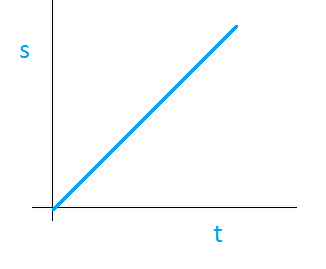• When the motion is non-uniform, the distance-time graph is not a straight line. It can be any curve.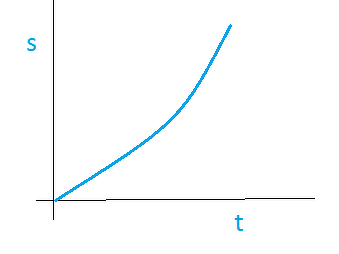Q2. What can you say about the motion of an object whose distance time-graph is a straight line parallel to the time axis?
Ans: If the distance-time graph is a straight line parallel to the time axis, the body is at rest.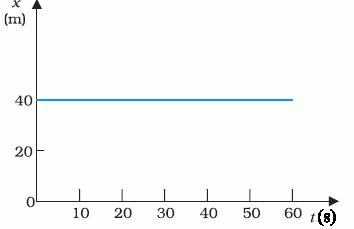Q3. What can you say about the motion of an object if its speed-time graph is a straight line parallel to the time axis?
Ans: If the speed-time graph is a straight line parallel to the time axis, the object is moving uniformly.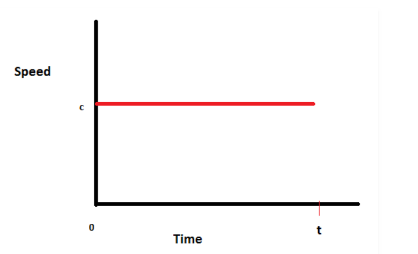Q4. What is the quantity which is measured by the area occupied below the velocity-time graph?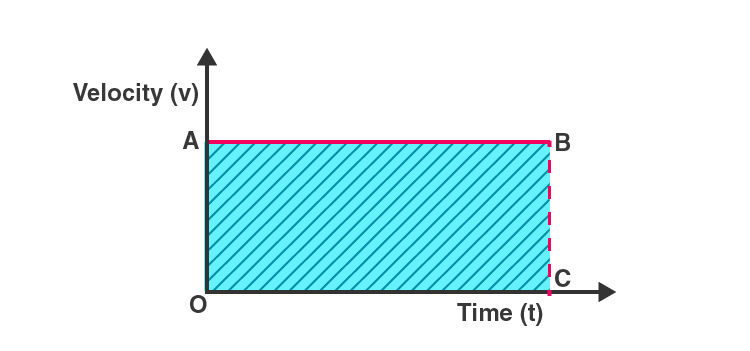Ans: The area under velocity-time graph = displacement of the body.

Page No. 109

Q1. A bus starting from rest moves with a uniform acceleration of 0.1 ms-2 for 2 minutes. Find
(a) the speed acquired.
(b) the distance travelled.
Ans: Initial velocity, u = 0 ms-1; Final velocity, v = ?; Acceleration, a = 0.1 ms-2; Time, t = 2 min = 120 s
(a) Speed, v = u + at = 0 + 0.1 x 120 = 12 ms-1
(b) Distance, s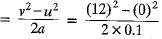= 720 m

Q2. A train is travelling at a speed of 90 km/h-1. Brakes are applied so as to produce a uniform acceleration of 0.5 ms-2. Find how far the train will go before it is brought to rest.
Ans: Initial speed of the train, u= 90 km/h = 25 m/s
Final speed of the train, v = 0 (finally the train comes to rest)
Acceleration = - 0.5 m s-2
According to third equation of motion:
v= u+ 2as (where, s is the distance covered by the train)
⇒ (0)= (25)+ 2 (- 0.5) s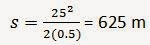The train will cover a distance of 625 m before it comes to rest.

Q3. A trolley, while going down an inclined plane, has an acceleration of 2 cm/s-2. What will be its velocity 3 s after the start?
Ans: Initial Velocity of trolley, u = 0 cms-1; Acceleration, a = 2 cms-2; Time, t = 3 s
We know that final velocity, v = u + at = 0 + 2 x 3 cms-1
Therefore, The velocity of train after 3 seconds = 6 cms-1

Q4. A racing car has a uniform acceleration of 4 ms2. What distance will it cover in 10 s after start?
Ans: Initial Velocity of the car, u = 0 ms-1; Acceleration, a = 4 m s-2; Time, t = 10 s
We know Distance, s = ut + (1/2) at2
Therefore, Distance covered by car in 10 second = 0 × 10 + (1/2) × 4 × 102 = (1/2) × 400 = 200 m

Q5. A stone is thrown in a vertically upward direction with a velocity of 5 m/s-1. If the acceleration of the stone during its motion is 10 m/s-2 in the downward direction, what will be the height attained by the stone and how much time will it take to reach there?
Ans: Given Initial velocity of stone, u = 5 ms-1
Downward of negative Acceleration, a = 10 ms-2
We know that: 2as = v2- u2

⇒ 0 = (5)+ 2 x(-10) x s
⇒ 0 = 25 - 20s
⇒ s = 25/20 = 1.25 m

Height attained by stone, s = 1.25 m

We know that: v = u + at

⇒ 0 = 5 + (–10) × t

⇒ 0 = 5 − 10t

⇒ t = 5/10 = 0.5 s
Thus, the stone will attain a height of 1.25 m and the time taken to attain the height is

0.5 s.

Page No. 112

Q1. An athlete completes one round of a circular track of diameter 200 m in 40 s. What will be the distance covered and the displacement at the end of 2 minutes 20 s?
Ans: Here, the diameter of the circular track = 200 m.
The radius of circular track, r = 100 m.
Let the athlete start moving from A, which is treated as a reference point.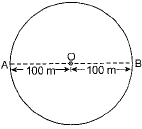The distance covered by the athletes in 40 s = circumference of the circle = 2πr = 2π x 100 m = 200π m
The distance traversed in one second = 200π/40 = 5π
Distance covered by athletes in 2 minutes and 20 seconds, i.e., 140 s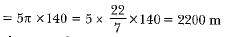Number of complete rounds done by the athlete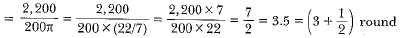Therefore, the final position of athletes at the end of 2 minutes and 20 seconds or just after three and a half rounds is B.
The displacement at the end of 2 minutes and 20 seconds = AB (diameter) = 200 m

Q2. Joseph jogs from one end A to the other end B of a straight 300 m road in 2 minutes 50 seconds and then turns around and jogs 100 m back to point C in another 1 minute. What are Joseph's average speeds and velocities in Jogging (a) from A to B and (b) from A to C?
Ans: Let Joseph jogs from A to B and back to C as shown.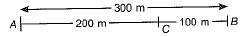(a) From A to B,
distance covered = displacement = 300 m
Time taken = 2 minutes 30 s = 150 s
∴ Average speed = average velocity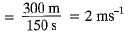(b) From A to C,
distance covered = 300 m + 100 m = 400 m
Total time = 150 s + 60 s = 210 s
Average speed = 400m/210s = 1.9 ms-1
However,
Displacement = 300 m - 100 m = 200 m, and Total time = 210 s
Average velocity = 200m/210s = 0.952 ms-1

Q3. Abdul while driving to school computes the average speed for his trip to be 20 kmh-1. On his return trip along the same route, there is less traffic and the average speed is 40 kmh-1. What is the average speed for Abdul's trip?
Ans: Let the distance between Abdul’s home and school be x.
Time taken by Abdul from home to school,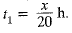Time taken by Abdul from school to home,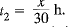Total time, t = t1 + t2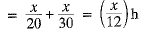Total distance, s = x + x = 2x km
Average speed =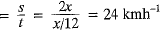Q4. A motorboat starting from rest on a lake accelerates in a straight line at a constant rate of 3.0 ms-2 for 8.0 s. How far does the boat travel during this time?
Ans: Here, u = 0 m/s, a = 3 ms-2 , t = 8 s
Using, s = ut + 1/2 at2
s = 0 x 8 + 1/2 x 3 x 82 = 96 m.

Q5. A driver of a car travelling at 52 kmh-1 applies the brakes and accelerates uniformly in the opposite direction. The car stops in 5s. Another driver going at 3 kmh-1 in another car applies his brakes slowly and stops in 10 s. On the same graph paper, plot the speed versus time graphs for the two cars. Which of the two cars travelled farther after the brakes were applied?

Ans: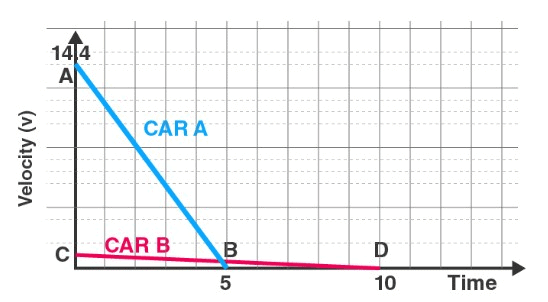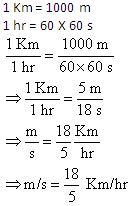Speed of car A = 52 km/h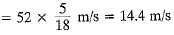Speed of car B = 3 km/h = 3 x 5/18 = 0.833 m/s
Distance covered by car 1 = area of ΔABC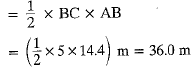Distance covered by car 2 = area of ΔBDE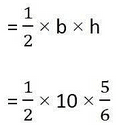= 4.166m
Thus, car 1 travelled farther after the brakes were applied.

Q6. Figure below shows the distance-time graph of three objects A, B and C. Study the graph and answer the following questions :
(a) Which of the three is travelling the fastest?
(b) Are all three ever at the same point on the road?
(c) How far has C travelled when B passes A?
(d) How far has B travelled by the time it passes C?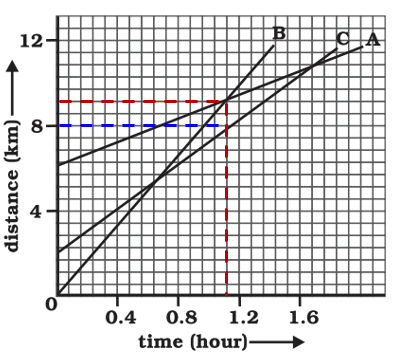Ans:

(a) since the slope of line B is the greatest, B is travelling at the fastest speed.

(b) since the three lines do not intersect at a single point, the three objects never meet at the same point on the road.

(c) since there are 7 unit areas of the graph between 0 and 4 on the Y axis, 1 graph unit equals 4/7 km.

Since the initial point of object, C is 4 graph units away from the origin, Its initial distance from the origin is 4*(4/7)km = 16/7 km

When A passes B, the distance between the origin and C is 8km

Therefore, total distance travelled by C in this time = 8 – (16/7) km = 5.71 km

(d) the distance that object B has covered at the point where it passes C is equal to 9 graph units.

Therefore, total distance travelled by B when it crosses C = 9*(4/7) = 5.14 km

Q7. A ball is gently dropped from a height of 20 m. If its velocity increases uniformly at the rate of 10 ms-2, with what velocity will it strike the ground? After what time will it strike the ground?
Ans: Initial velocity of ball, u = 0 (as it is dropped)
Height, h = 20 m, Acceleration, a = 10 ms-2,
Final velocity, v = ?, Time, t = ?
v2 = u2 + 2 as
Here, s = h
∴ v2 = 0 + 2 x 10 x 20 = 40
or Final velocity, v = 20 ms-1
v = u + at
20 = 0 + 10t
∴ t = 20/10
or time, t = 2 s

Q8. The speed-time graph for a car is shown in figure below.
(a) Find how far does the car travel in the first 4 seconds. Shade the area on the graph that represents the distance travelled by the car during the period.
(b) Which part of the graph represents uniform motion of the car?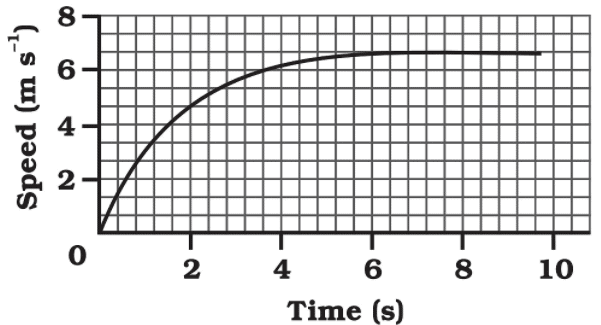Ans:
(a)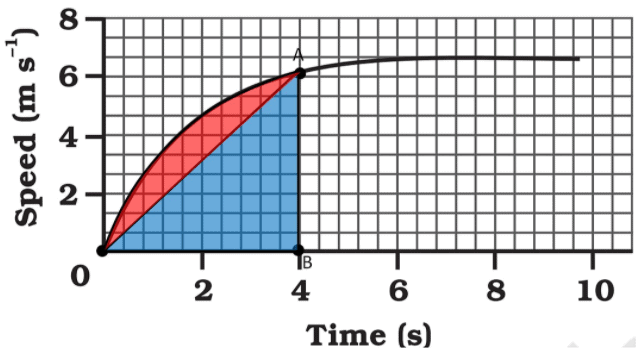In velocity-time graph,

Distance = Area of v-t graph

Here we approximate the area using area of triangle,

Distance travelled = Area of triangle AOB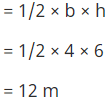The shaded area, which is equal to 1/2 × 4 × 6 = 12 m represents the distance travelled by the car in the first 4 s.
(b)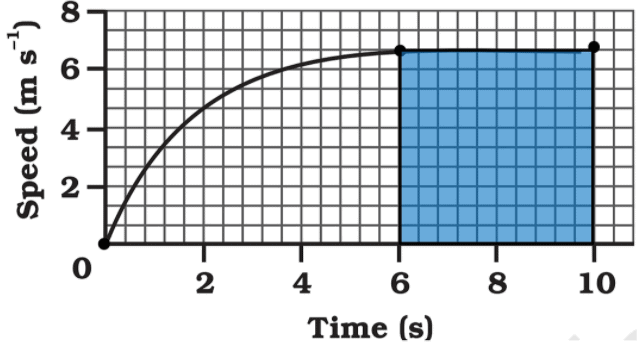The part of the graph in red colour between time 6 s to 10 s represents uniform motion of the car.

Q9. State which of the following situations are possible and give an example for each of these.
(a) An object with a constant acceleration but with zero velocity.
(b) An object moving in a certain direction with acceleration in the perpendicular direction.
(c) an object moving with acceleration but with uniform speed.
Ans:
(a) When an object is thrown upwards, it comes to a momentary rest at the highest point. Thus velocity is zero, but the acceleration due to the gravitational pull of the earth still acts upon it.
(b) In a uniform circular motion, the speed remains constant, but there is varying velocity as it changes its direction, so there always be acceleration which is given by centripetal force.
(c) When an object is thrown in the forward direction, then during its motion in the horizontal direction, the acceleration due to the gravity of earth acts in the vertically downward direction.

Q10. An artificial satellite is moving in a circular orbit of a radius of 42250 km. Calculate its speed if it takes 24 hours to revolve around the earth.
Ans: Satellite completes one round in 24 hours
t = 24 h = 24 x 60 min. = 24 x 3600 s
Angle traversed by the satellite in completing one round = 2π
Linear speed of satellite = 2πr / t

= (2 x 3.14 x 42250 km) / (24 x 3600 s)

= (6.28 x 42250 km) / (24 x 3600 s) (∵ π = 3.14)
= 3.1 km/s

The document NCERT Solutions - Motion, Class 9, Science | EduRev Notes is a part of the Class 9 Course NCERT Textbooks (Class 6 to Class 12).
All you need of Class 9 at this link: Class 9Use Code STAYHOME200 and get INR 200 additional OFF Use Coupon Code
All Tests, Videos & Notes of Class 9: Class 9

### Top Courses for Class 9## NCERT Textbooks (Class 6 to Class 12)

955 docs|585 tests

### Top Courses for Class 9Track your progress, build streaks, highlight & save important lessons and more!

,

,

,

,

,

,

,

,

,

,

,

,

,

,

,

,

,

,

,

,

,

,

,

,

,

,

,

;